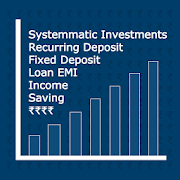# Financial Calculators, Banking

### By DailyMobApps

Take control and grasp your private finances through the use of the Financial Calculators / Banking calculator app

This includes Equated monthly Instalment (EMI Calculator), Systematic Investment Plan (SIP calculator), Recurring Deposit Interest calculators (RD Calculator), Fixed Deposit (FD calculator)

Financial Calculators app supports under calculators

☆ EMI calculator ( Calculate EMIs for Home loan, Car loan, Personal Loan, Education loan)

☆ Fixed deposit Interest calculator

☆ Recurring deposit Interest calculator

☆ SIP returns calculator

☆ SIP period calculator

☆ SIP goal calculator

☆ Interest rate calculator

☆ Home loan eligibility calculator

App is free, offline.

Financial Calculators, Banking Tags